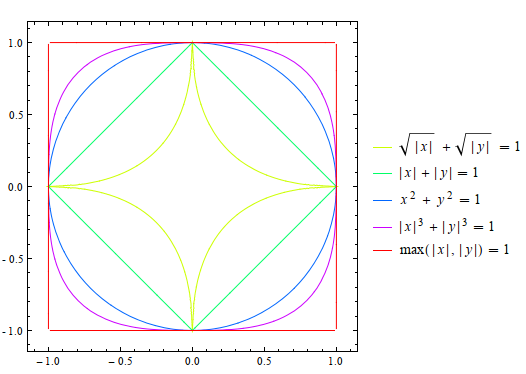## Unit circle under different norms

There are many ways to calculate distance in $R^n$, one family of them are called p-norms. (norm is the distance to the origin.)

\begin{align*} D &= (\sum |x_i|^p)^{1/p} \end{align*}

Among them 1-norm is called the Taxicab-norm, 2-norm the Euclidean norm. Below is a plot of unit circles under different norms. Note how the curvature changes with p!Unit circle under different norms (mathematica code)
Click to toggle code (may have to try twice)
ContourPlot[{
Abs[x]^(1/2)+Abs[y]^(1/2)==1,
Abs[x] + Abs[y] == 1,
x^2+y^2==1,
Abs[x]^3+Abs[y]^3==1,
Max[Abs[x], Abs[y]] == 1
},
{x, -1.1, 1.1}, {y, -1.1, 1.1},
ContourLabels -> All,
PlotLegends -> "Expressions",
ContourStyle -> {Hue[1/5], Hue[2/5], Hue[3/5], Hue[4/5], Hue[5/5]}
]